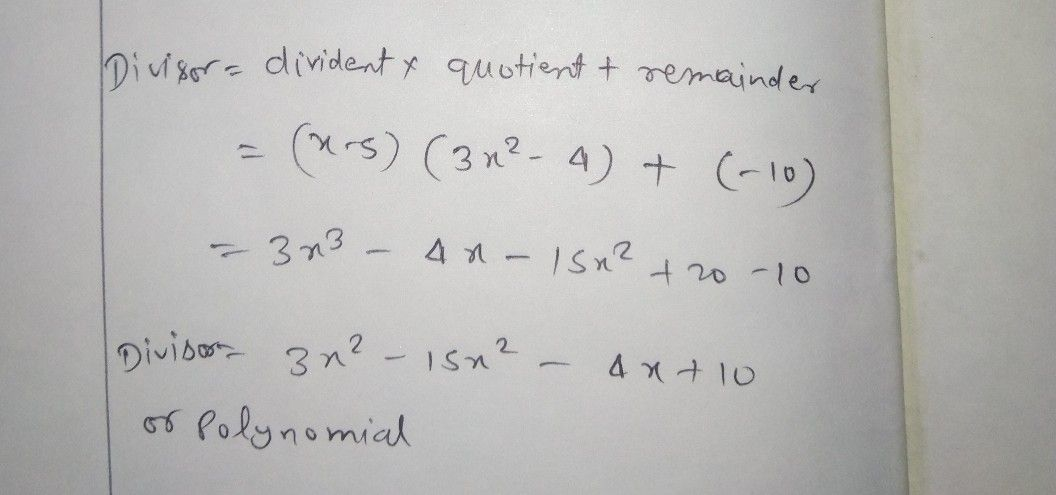Symbol
ProblemRead and $ana|yze$ the given problem. Write your answer on a separate sheet of paper. Show your complete solution. $1.$ When a polynomial is divided by $x-5,$ the quotient is $3x^{2}-4$ and the remainder is $s-10.$ Find the polynomial.